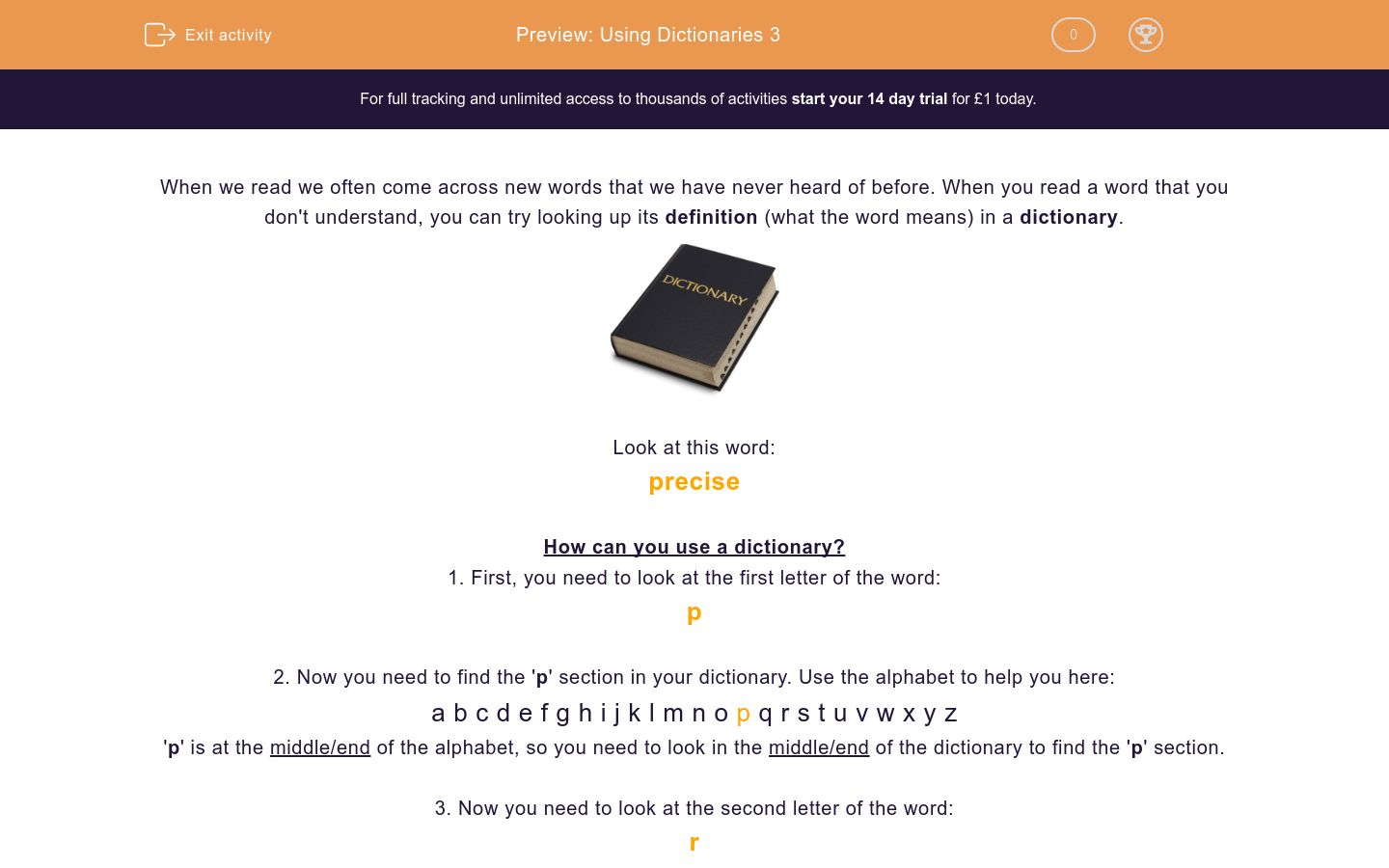# Using Dictionaries 3

In this worksheet, students will be asked to read new words and find their meanings in a dictionary.Key stage:  KS 2

Curriculum subtopic:   Use Dictionaries

Difficulty level:### QUESTION 1 of 10

When we read we often come across new words that we have never heard of before. When you read a word that you don't understand, you can try looking up its definition (what the word means) in a dictionary.Look at this word:

precise

How can you use a dictionary?

1. First, you need to look at the first letter of the word:

p

2. Now you need to find the 'p' section in your dictionary. Use the alphabet to help you here:

a b c d e f g h i j k l m n o p q r s t u v w x y z

'p' is at the middle/end of the alphabet, so you need to look in the middle/end of the dictionary to find the 'p' section.

3. Now you need to look at the second letter of the word:

r

4. Now you need to find the 'pr' section. Use the alphabet to help you here:

a b c d e f g h i j k l m n o p q r s t u v w x y z

'r' is at the middle/end of the alphabet, so you need to look for 'pr' words in the middle/end of the 'p' section.

5. Now you need to look at the third letter of the word:

e

6. Now you need to find the 'pre' section. Use the alphabet to help you here:

a b c d e f g h i j k l m n o p q r s t u v w x y z

'e' is in the beginning of the alphabet, so you need to look for 'pre' words in the beginning of the 'pr' section.

7. Now look down the 'pre' word section, until you find:

precise

You have now hopefully found its definition is:

Something that is exact in every detail.

In this activity, you will need to use your dictionary to find out the definitions of some new words.

Remember, the definitions in your dictionary may have a slightly different explanation, but the meaning should be the same.

---- OR ----